# Use the Formula Editor

ExamSoft includes the WIRIS/Math Type formula editor. Using this editor, you can create formulas and equations for any kind of content: mathematics, physics, and the sciences.

Note:
For detailed instructions, go to these resources on the Math Type help site:

## Procedure

1. Within the question toolbar, stem, or answer choice, select the Formula Editor button (square root symbol).

Enterprise Portal: Location of Formula Editor button in question stem and answer choices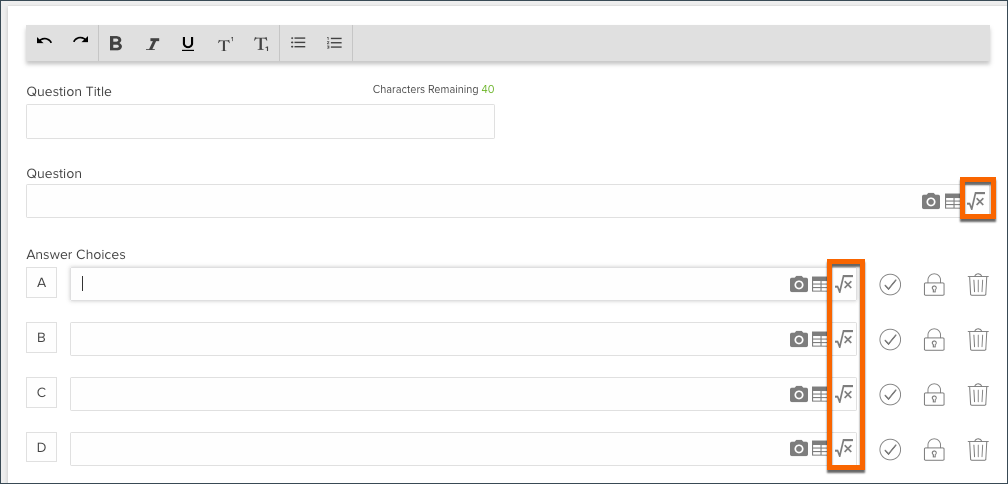Enterprise Portal: Location of Formula Editor button in toolbarLegacy Portal: Location of Formula Editor button2. At the top of the WIRIS editor, select the tab for the type of formula that you want to add.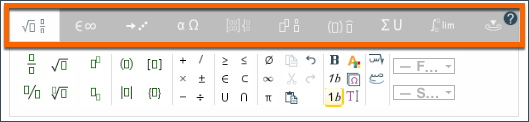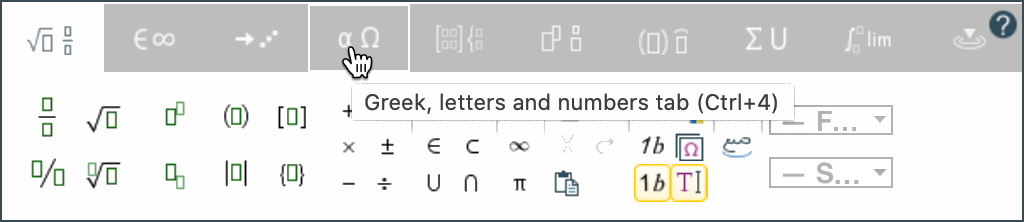3. Create your equation by selecting buttons, typing characters, or using the touchscreen option (see the Tips below this procedure).
4. When you're ready to insert your formula, select the Insert or Accept button (in the lower right corner of the editor).

Tips:

• For detailed information about the formula editor, select the Help button (in the top right corner of the toolbar).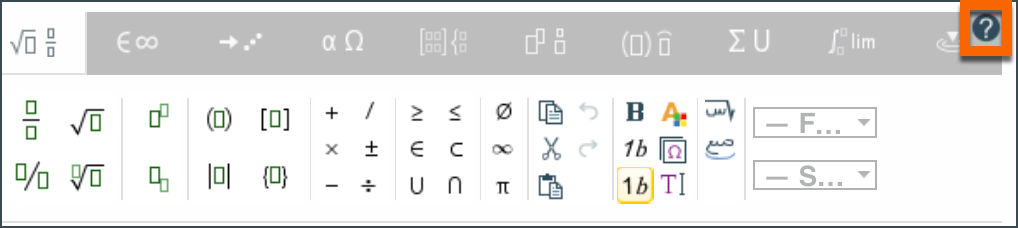Example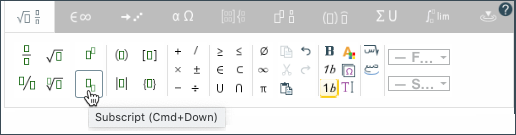• To use your touchscreen and/or mouse/keyboard to draw the formula, select the touchscreen button (on the right).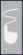Within the touchscreen area, you can use these options:

• Select the Undo button if you make a mistake.
• Use the eraser icon to fix a small mistake.
• To reset the entire equation, select the trash can icon
• To return to the default view, select the keyboard button (in the lower left corner).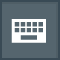For more information, see "Handwritten Input" article on the Math Type help site.

• To return to regular text after entering subscript or superscript, press the right-arrow key.
• To navigate between the layers of a fraction, use the up- and down-arrow keys.
• To find additional characters that are not shown on the General toolbar, select the Insert special characters button (next to the Italic button).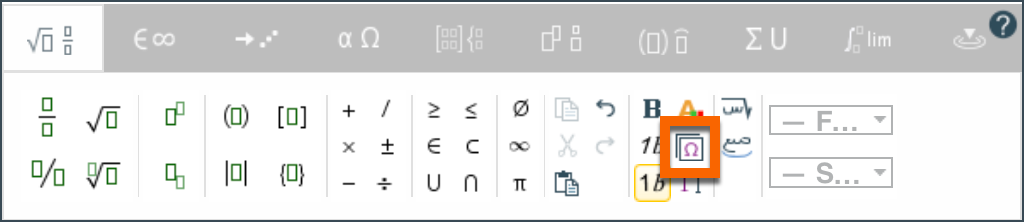• If you need to edit a formula after inserting it, simply click on it to reopen the editor.
• For tips about using the formula editor with screen reader software, your keyboard, and other assistive technology, see the "Accessibility" article on the Math Type help site.
• For more help with the WIRIS editor, see the example section of this article or go to the Math Type WIRIS tutorials

## Example

This example walks you through the steps to create a Multiple Choice question that includes formulas in the question stem and the answer choices.

Question Stem
As shown below, we want to use a formula within the question stem: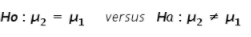1. Create a new Multiple Choice question.
2. In the question stem area, select the Formula button.
3. Use your keyboard to type the characters, spaces, and colon at the beginning of the equation.
4. To enter the first Mu and the subscript 2:
1. Select the Greek tab, and then select the Mu button.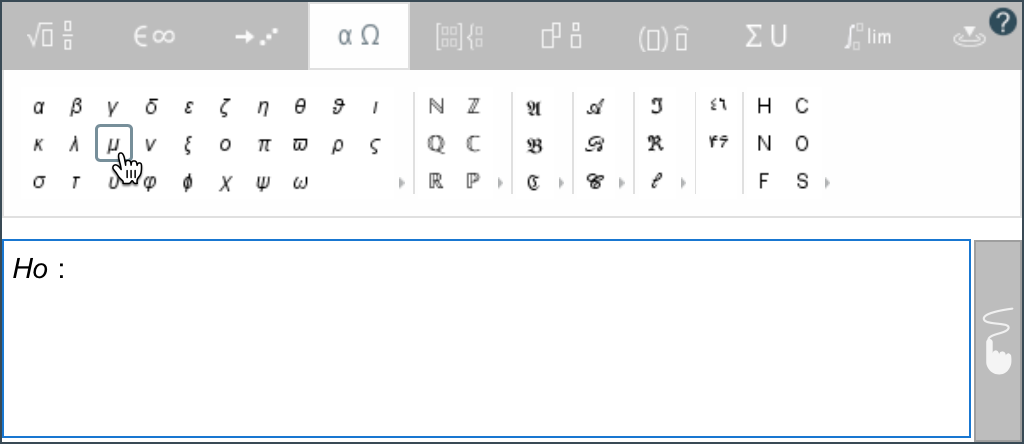2. Select the General tab, and then select the Subscript button.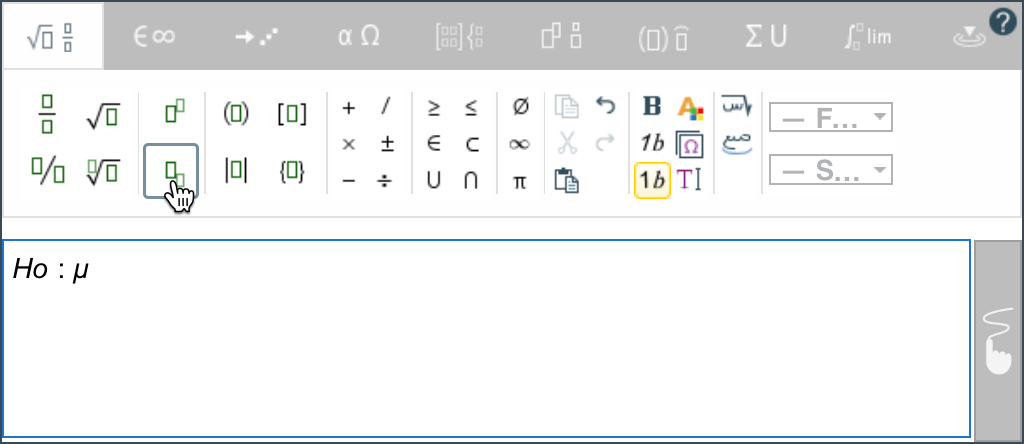5. Press the right-arrow key to move the cursor out of the subscript area.
6. Type the equal-to symbol by using your keyboard.
7. To enter the next Mu and subscript, simply copy and paste the first one, and then change the subscript number to 1.
8. Press the right-arrow key, and then continue entering the equation.
9. To enter the not-equal-to symbol:
1. Select the Insert special characters button.
2. Scroll about halfway down, and then select the not-equal-to button.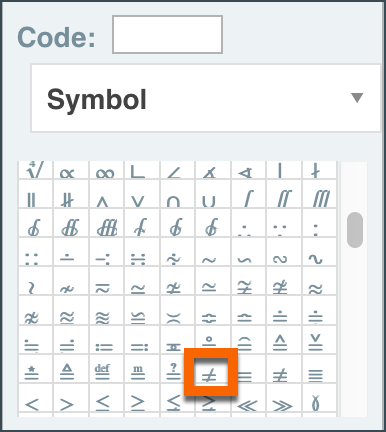Tip: To find a symbol more easily, enter its decimal or hexadecimal code. You can look up these codes by using a resource such as UnicodeLookup.com.

10. Finish entering the equation, and then select Insert.

For the first answer choice, we want to enter this equation: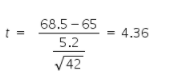1. Select the Formula button for Answer Choice A.
2. Use your keyboard to enter the letter t and the equal-to symbol.
3. Select the Scripts and layout tab.
4. Select the Big fraction button.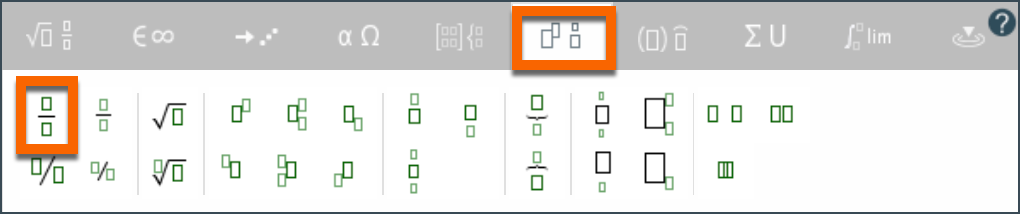5. Click in the numerator area, and use your keyboard to enter 68.5-65.
6. To enter the fraction in the denominator:
1. Click in the denominator area, and then select the Small fraction button.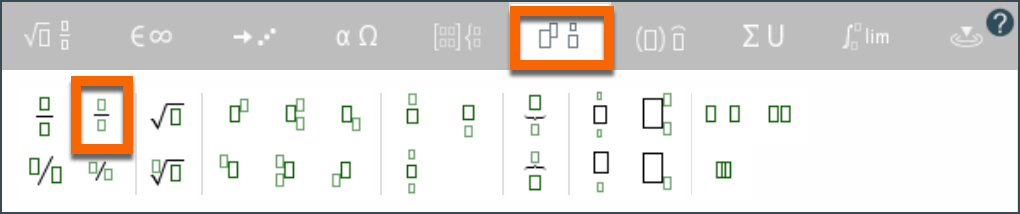2. Enter the number 5.2 in the numerator.
3. In the denominator, select the Square root button, and then enter 42.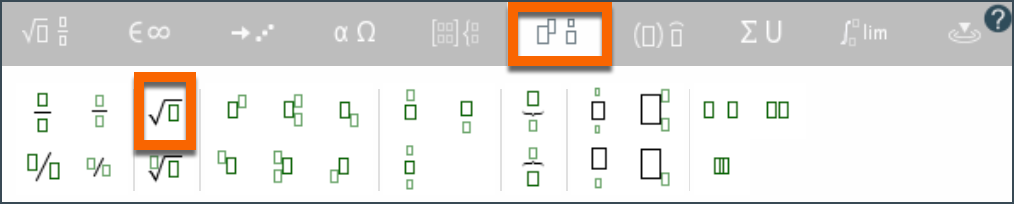7. Press the right-arrow key until the cursor is on the right side of the big fraction and the cursor height matches the height of the big fraction.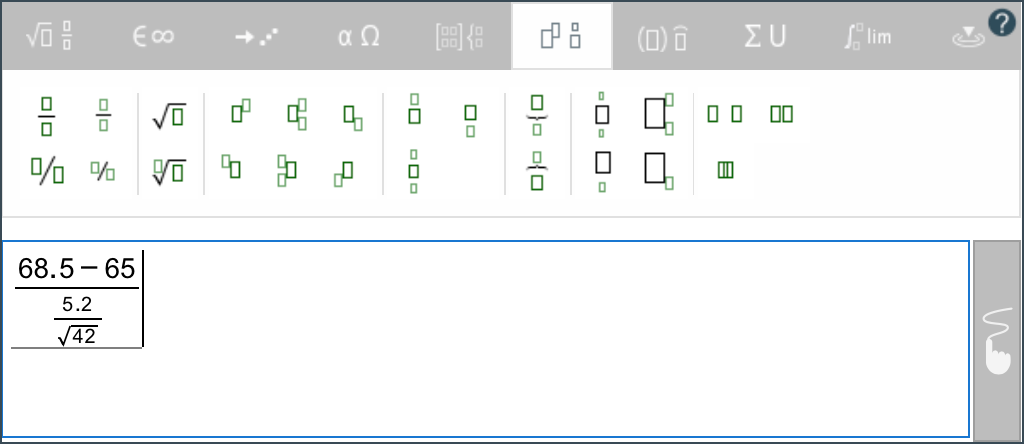8. Use your keyboard to enter the rest of the equation.
9. Finish entering the equation, and then select Insert.

For the second answer choice, we want to enter this equation: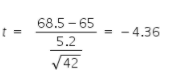1. Click on the formula in Answer Choice A to reopen the editor.
2. Drag your mouse across the enter equation to select it, and then select the Copy button.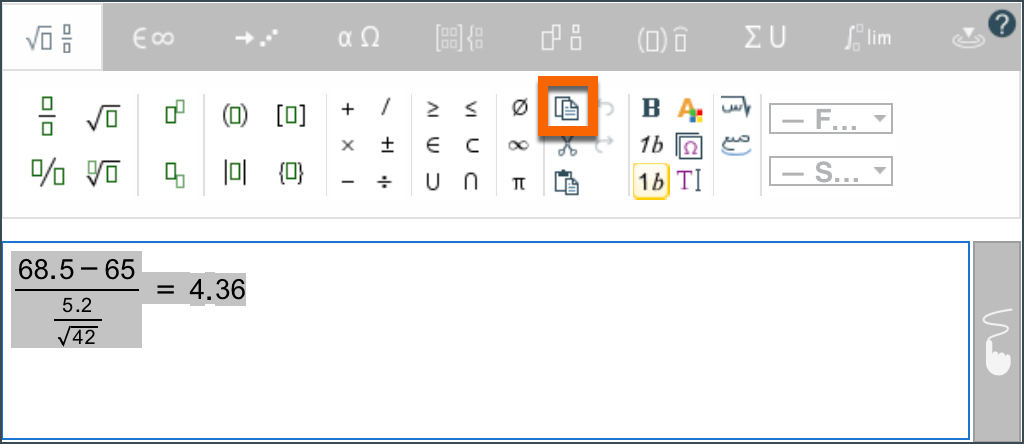3. Select Cancel to close Answer Choice A.
2. Select the Formula button for Answer Choice B.
3. Select the Paste button to paste the copied equation.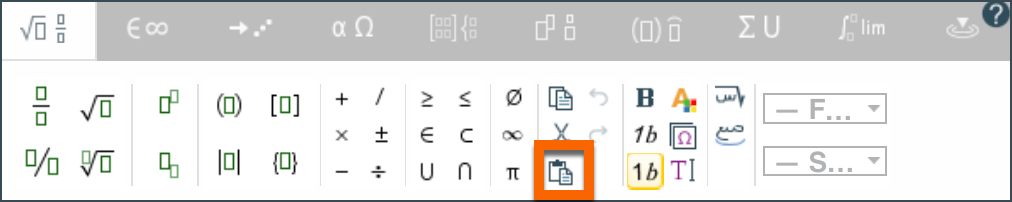4. Insert a minus sign to change 4.36 to -4.36, as shown in the example.
5. Select Insert.

For the third answer choice, we want to enter this equation: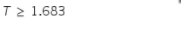You can use your keyboard to type the letter and numbers. You'll find the Greater-than-or-equal-to button near the center of the toolbar on the General tab.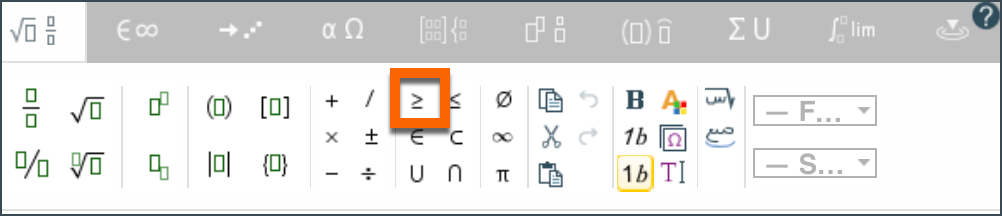For the final answer choice, we want to enter this equation: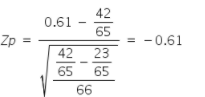This example uses techniques that you learned in the previous examples. Use the Big fraction, Small fraction, and Square root buttons to enter the equation.

Tip: Use the arrow keys to navigate through the various levels of fractions.

• Up-Arrow: Move the cursor into the numerator.
• Down-Arrow: Move the cursor into the denominator.
• Left: Move the cursor to the left of the current object.
• Right: Move the cursor to the left of the current object.